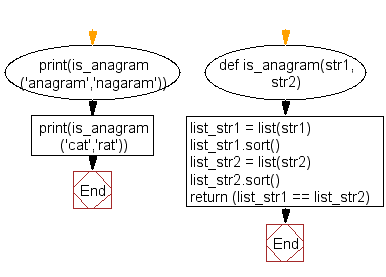﻿ Python: Check if a given string is an anagram of another given string - w3resource

# Python Challenges: Check if a given string is an anagram of another given string

## Python Challenges - 1: Exercise-26 with Solution

Write a Python program to check if a given string is an anagram of another given string.

According to Wikipedia an anagram is direct word switch or word play, the result of rearranging the letters of a word or phrase to produce a new word or phrase, using all the original letters exactly once; for example, the word anagram can be rearranged into nag-a-ram.

Explanation:Sample Solution:

Python Code:

``````def is_anagram(str1, str2):
list_str1 = list(str1)
list_str1.sort()
list_str2 = list(str2)
list_str2.sort()

return (list_str1 == list_str2)

print(is_anagram('anagram','nagaram'))
print(is_anagram('cat','rat'))
```
```

Sample Output:

```True
False
```

Flowchart:Python Code Editor: